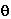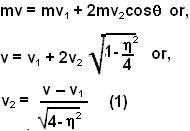## Thursday, June 5, 2008

### Irodov Problem 1.176As shown in the figure, after the collision, balls B and C will move at angle. Suppose that after collision, the velocity of ball A becomes v1. Since ball A collides symmetrically with balls B and C the final direction of motion of ball A will be along the same direction as its original motion (the vertical components of the normal reactions from balls B and C during collision will cancel each other out) as shown in the figure.

Since there are no external forces on the system momentum before and after collision must be conserved. Conserving the component of momentum along the vertical direction, its is clear velocities of both balls B and C should be the same (since the initial value of momentum along the vertical direction is 0). Let the final velocity of balls B and C be v2.

Conserving the momentum along the horizontal direction we have,Since it is an elastic collision the energy before and after collision must be conserved,So ifthen the value of v1 will be negative i.e. it will recoil back. Ifthe ball will stop since v1=0. At greater values, the ball will move on since v1>0.